# PSEB 6th Class Maths Solutions Chapter 12 Perimeter and Area Ex 12.1

Punjab State Board PSEB 6th Class Maths Book Solutions Chapter 12 Perimeter and Area Ex 12.1 Textbook Exercise Questions and Answers.

## PSEB Solutions for Class 6 Maths Chapter 12 Perimeter and Area Ex 12.1

1. Find the perimeter of the following shapes:

Question (i)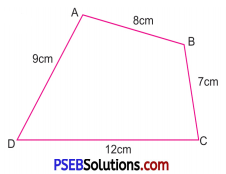Solution:
Perimeter = AB + BC + CD + DA
= 8 cm + 7 cm + 12 cm + 9 cm
= 36 cmQuestion (ii)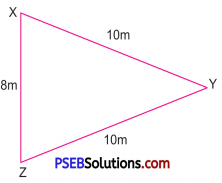Solution:
Perimeter = XY + YZ + ZX
= 10m + 10m + 8m
= 28m

Question (iii)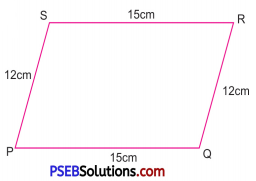Solution:
Perimeter = PQ + QR + RS + SP
= 15 cm + 12 cm + 15 cm + 12 cm
= 54 cm

Question (iv)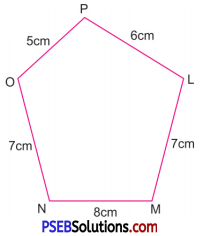Solution:
Perimeter = MN + NO + OP + PL + LM
= 8 cm + 7 cm + 5 cm + 6 cm + 7 cm
= 33 cmQuestion (v)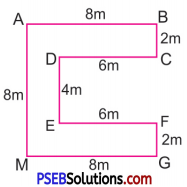Solution:
Perimeter = AB + BC + CD + DE + EF + FG + GM + MA
=8m + 2m + 6m + 4m + 6m + 2m + 8m + 8m
= 44 m

Question (vi)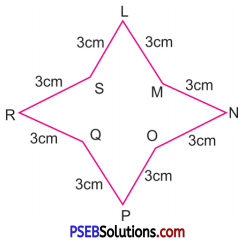Solution:
Perimeter = LM + MN + NO + OP + PQ + QR + RS + SL
= 3 cm + 3 cm + 3 cm + 3 cm + 3 cm + 3 cm + 3 cm + 3 cm
= 24 cm

2. Find the perimeter of the triangle with sides:

Question (i)
5 cm, 6 cm and 7 cm
Solution:
Perimeter of a triangle
= Sum of lengths of its sides
= 5 cm + 6 cm + 7 cm = 18 cmQuestion (ii)
10 m, 12 m, 18 m
Solution:
Sides of triangle
= 10 m, 12 m, 18 m
∴ Perimeter of a triangle
= Sum of lengths of its sides
= 10 m + 12 m + 18 m = 40 m

Question (iii)
4.6 cm, 3.2 cm and 5.8 cm.
Solution:
Sides of triangle
= 4.6 cm, 3.2 cm and 5.8 cm
∴ Perimeter of triangle
= Sum of lengths of its sides
= 4.6 cm + 3.2 cm + 5.8 cm
= 13.6 cm

3. Find the perimeter of an isosceles triangle with 15 cm as length of equal side and 18 cm as base.
Solution:
Sides of isosceles triangle = 15 cm, 15 cm, 18 cm
Area of isosceles triangle = Sum of lengths of its sides
= (15 + 15 + 18) cm = 48 cm4. Find the perimeter of a square with side:

Question (i)
16 cm
Solution:
Side of square = 16 cm
∴ Perimeter of square = 4 × side
= 4 × 16 cm
= 64 cm

Question (ii)
4.8 mm
Solution:
Side of square = 4.8 mm
∴ Perimeter of square = 4 × side
= 4 × 4.8 mm
= 19.2 mm

Question (iii)
125 cm
Solution:
Side of square = 125 cm
∴ Perimeter of square = 4 × side
= 4 × 125 cm
= 500 cm

Question (iv)
45 m
Solution:
Side of square = 45 m
∴ Perimeter of square = 4 × side
= 4 × 45 m
= 180 mQuestion (v)
39 cm.
Solution:
Side of square = 39 cm
∴ Perimeter of square = 4 × side
= 4 × 39 cm
= 156 cm

5. Find the perimeter of a rectangle with:

Question (i)
Length 20 m and breadth 15 m
Solution:
Length of rectangle = 20 m
Breadth of rectangle = 15 m
∴ Perimeter of rectangle = 2 × (length + breadth)
= 2 × (20 + 15) m
= 2 × 35 m
= 70 m

Question (ii)
Length 25 m and breadth 35 m
Solution:
Length of rectangle = 25 m
Breadth of rectangle = 35 m
∴ Perimeter of rectangle = 2 × (length + breadth)
= 2 × (25 + 35) m
= 2 × 60 m
= 120 m

Question (iii)
Length 40 cm and breadth 28 cm
Solution:
Length of rectangle = 40 cm Breadth of rectangle = 28 cm
∴ Perimeter of rectangle = 2 × (length + breadth)
= 2 × (40 + 28) cm
= 2 × 68 cm
= 136 cmQuestion (iv)
Length 18.3 cm and breadth 6.8 cm
Solution:
Length of rectangle = 18.3 cm
Breadth of rectangle = 6.8 cm
∴ Perimeter of rectangle
= 2 × (length + breadth)
= 2 × (18.3 + 6.8) cm
= 2 × 25.1 cm = 50.2 cm

Question (v)
Length 0.125 m and breadth 15 cm.
Solution:
Length of rectangle
= 0.125 m = 12.5 cm
Breadth of rectangle = 15 cm
∴ Perimeter of rectangle = 2 × (length + breadth)
= 2 × (12.5 + 15) cm
= 2 × 27.5 cm
= 55 cm

6. Find the perimeter of a regular hexagon with side:

Question (i)
5 cm
Solution:
Side of a regular hexagon = 5 cm
Perimeter of a regular hexagon = 6 × side
= 6 × 5 cm
= 30 cmQuestion (ii)
12 cm
Solution:
Side of a regular hexagon = 12 cm
Perimeter of a regular hexagon = 6 × side
= 6 × 12 cm
= 72 cm

Question (iii)
7.2 cm.
Solution:
Side of a regular hexagon = 7.2 cm
Perimeter of a regular hexagon = 6 × side
= 6 × 7.2 cm
= 43.2 cm

7. Find the perimeter of an equilateral triangle with side:

Question (i)
10 cm
Solution:
Side of an equilateral triangle
= 10 cm
∴ Perimeter of an equilateral triangle = 3 × side
= 3 × 10 cm
= 30 cm

Question (ii)
8 m
Solution:
Side of an equilateral triangle = 8 m
∴ Perimeter of an equilateral triangle = 3 × side
= 3 × 8 m
= 24 mQuestion (iii)
24 m
Solution:
Side of an equilateral triangle = 24 m
∴ Perimeter of an equilateral triangle = 3 × side
= 3 × 24 m
= 72 m

Question (iv)
5.6 m
Solution:
Side of an equilateral triangle = 5.6 m
∴ Perimeter of an equilateral triangle = 3 × side
= 3 × 5.6 m
= 16.8 m

Question (v)
12.1 cm.
Solution:
Side of an equilateral triangle = 12.1 cm
∴ Perimeter of an equilateral triangle = 3 × side
= 3 × 12.1 cm
= 36.3 cm8. If the perimeter of a triangle is 48 cm and two sides are 12 cm and 17 cm. Find the third side.
Solution:
Perimeter of a triangle = 48 cm Sum of length of two sides = (12 + 17) cm = 29 cm
∴ Third side = 48 cm – 29 cm
= 19 cm

9. Find the side of an equilateral triangle, if the perimeter is:

Question (i)
45 cm
Solution:
Given perimeter of an equilateral triangle = 45 cm
Perimeter of an equilateral triangle = 3 × (side of the triangle)
⇒ 45 cm = 3 × side
⇒ Side of the triangle
= 15cm

Question (ii)
69 mm
Solution:
Given perimeter of an equilateral triangle = 69 mm
Perimeter of an equilateral triangle = 3 × (side of the triangle)
⇒ 69 = 3 × (side of the triangle)
⇒ Side of the triangle = $$\frac{69 \mathrm{~mm}}{3}$$
= 23 mmQuestion (iii)
117 cm.
Solution:
Given perimeter of an equilateral triangle = 117 cm
Perimeter of an equilateral triangle = 3 × (side of the triangle)
⇒ 117 = 3 × (side of the triangle)
⇒ Side of the triangle = $$\frac{117 \mathrm{~cm}}{3}$$
= 39 cm

10. Find the side of a square if the perimeter is:

Question (i)
52 cm
Solution:
Given Perimeter of a square = 52 cm
Perimeter of a square = 4 × (side of square)
⇒ Side of square
= $$\frac{\text { perimeter of a square }}{\text { 4 }}$$
= $$\frac{52 \mathrm{~cm}}{4}$$
= 13 cm

Question (ii)
60 cm
Solution:
Given perimeter of a square = 60 cm
Side of a square
= $$\frac{\text { perimeter of a square }}{\text { 4 }}$$
= $$\frac{60 \mathrm{~cm}}{4}$$
= 15 cm

Question (iii)
112 cm.
Solution:
Given perimeter of a square = 112 cm
Side of a square
= $$\frac{\text { perimeter of a square }}{\text { 4 }}$$
= $$\frac{112 \mathrm{~cm}}{4}$$
= 28 cm11.

Question (i)
The perimeter of rectangular field is 260 m. If its length is 80 m then find its breadth.
Solution:
Given perimeter of rectangular field = 260 m
and Length of the rectangular field = 80 m
∴ Perimeter of rectangular field = 2 × (length + breadth)
⇒ 260 = 2 × (80 + breadth)
⇒ $$\frac {260}{2}$$ = 80 + breadth
⇒ 80 + breadth = 130
⇒ breadth = 130 – 80 = 50 m
Hence breadth of rectangular field = 50 m

Question (ii)
The perimeter of a rectangular garden is 140 m. If its breadth is 45 m then find its length.
Solution:
Given perimeter of rectangular garden = 140 m
and breadth of rectangular garden = 45 m
∴ Perimeter of rectangular garden = 2 × (length + breadth)
⇒ 140 = 2 × (length + 45)
⇒ $$\frac {260}{2}$$ = length + 45
⇒ length = 70 – 45 = 25 m
Hence length of rectangular garden = 25 m

Question (iii)
The perimeter of a rectangle is 114 cm. If its length is 32 cm then find its breadth.
Solution:
Given perimeter of rectangle = 114 cm
and length of rectangle = 32 cm
∴ Perimeter of rectangle
= 2 × (length + breadth)
⇒ 114 = 2 × (32 + breadth)
⇒ $$\frac {114}{2}$$ = 32 + breadth
⇒ breadth = 57 – 32 = 25 cm
Hence breadth of rectangle = 25 cm12. The side of a triangular field are 15 m, 20 m and 18 m. Find the total distance travelled by a boy in taking 2 complete rounds of this field.
Solution:
Sides of a triangular fields = 15 m, 20 m and 18 m
Distance covered in one round of a triangular field = Perimeter of rectangular field = Sum of the length of the sides of a rectangular field
= 15 m + 20 m + 18 m
= 53 m
∴ Distance covered in taking 2 complete rounds of this field
= 2 × 53 m
= 106 m

13. Find the cost of fencing a square field of side 26 m at the rate of ₹ 3 per metre.
Solution:
Given side of the square field = 26 m
∴ Perimeter of the square field = 4 × side
= 4 × 26 m = 104 m
Perimeter of fencing = 104 m
Cost of 1 m of fencing = ₹ 3
Cost of 104 m of fencing
= 104 × ₹ 3
= ₹ 31214. Mani runs around a square park of side 75 m. Kush runs around a rectangular park of length 60 m and breadth 45 m. Who covers less distance?
Solution:
Side of a square park = 75 m
Perimeter of square park = 4 × (side)
= 4 × (75 m) = 300 m
∴ Distance covered by Mani = 300 m
Length of rectangular park = 60 m
Breadth of rectangular park = 45 m
Perimeter of rectangular park = 2 (length + breadth)
= 2 × (60 + 45) m
= 2 × (105) m
= 210 m
∴ Distance covered by Kush = 210 m
Kush covers less distance.

15. Find the cost of framing a rectangular whiteboard with length 240 cm and breadth 150 cm at the rate of ₹ 6 per cm.
Solution:
Length of rectangular white board = 240 cm
Breadth of rectangular white board = 150 cm
Perimeter of rectangular white board = 2 × (length + breadth)
= 2 × (240 + 150) cm
= 2 × (390) cm
= 780 cm
Cost of fencing 1 cm = ₹ 6 × 780
= ₹ 468016. If length of a rectangle is ‘a’ units and breadth is 5 units. Find the perimeter of the rectangle.
Solution:
Given length of rectangle = ‘a’ units,
and Breadth of rectangle = 5 units
Perimeter of rectangle = 2 × (length + breadth)
= 2 × (a + 5) units
= 2 (a + 5) units

17. Fill in the blanks:

Question (i)
The sum of lengths of all sides of a polygon is called ……………. .
Solution:
perimeter

Question (ii)
Perimeter of Square = ……………. × side.
Solution:
4

Question (iii)
Perimeter of Rectangle = 2 × (………. +………) .
Solution: